CAT  >  Test: Problems On Trains- 1

# Test: Problems On Trains- 1

Test Description

## 10 Questions MCQ Test Quantitative Aptitude (Quant) | Test: Problems On Trains- 1

Test: Problems On Trains- 1 for CAT 2022 is part of Quantitative Aptitude (Quant) preparation. The Test: Problems On Trains- 1 questions and answers have been prepared according to the CAT exam syllabus.The Test: Problems On Trains- 1 MCQs are made for CAT 2022 Exam. Find important definitions, questions, notes, meanings, examples, exercises, MCQs and online tests for Test: Problems On Trains- 1 below.
Solutions of Test: Problems On Trains- 1 questions in English are available as part of our Quantitative Aptitude (Quant) for CAT & Test: Problems On Trains- 1 solutions in Hindi for Quantitative Aptitude (Quant) course. Download more important topics, notes, lectures and mock test series for CAT Exam by signing up for free. Attempt Test: Problems On Trains- 1 | 10 questions in 10 minutes | Mock test for CAT preparation | Free important questions MCQ to study Quantitative Aptitude (Quant) for CAT Exam | Download free PDF with solutions
 1 Crore+ students have signed up on EduRev. Have you?
Test: Problems On Trains- 1 - Question 1

### A train overtakes two persons who are walking in the same direction to that of the train at 2 kmph and 4 kmph and passes them completely in 9 and 10 seconds respectively. What is the length of the train?

Detailed Solution for Test: Problems On Trains- 1 - Question 1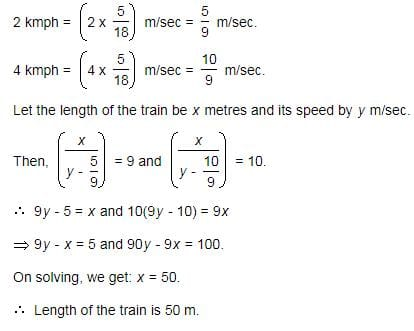Test: Problems On Trains- 1 - Question 2

### A train moves past a post and a platform 264 m long in 8 seconds and 20 seconds respectively. What is the speed of the train?

Detailed Solution for Test: Problems On Trains- 1 - Question 2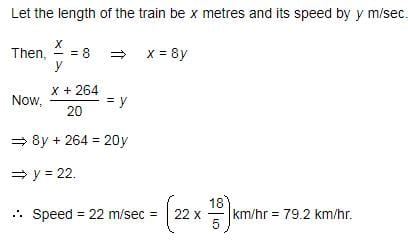Test: Problems On Trains- 1 - Question 3

### Two trains, one from P to Q and the other from Q to P, start simultaneously. After they meet, the trains reach their destinations after 9 hours and 16 hours respectively. The ratio of their speeds is

Detailed Solution for Test: Problems On Trains- 1 - Question 3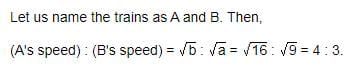Test: Problems On Trains- 1 - Question 4

A train runs at the speed of 72 kmph and crosses a 250 m long platform in 26 seconds. What is the length of the train?

Detailed Solution for Test: Problems On Trains- 1 - Question 4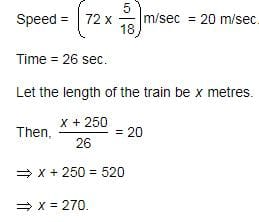Test: Problems On Trains- 1 - Question 5

Two trains having equal lengths, take 10 seconds and 15 seconds respectively to cross a post. If the length of each train is 120 meters, in what time (in seconds) will they cross each other when traveling in opposite direction?

Detailed Solution for Test: Problems On Trains- 1 - Question 5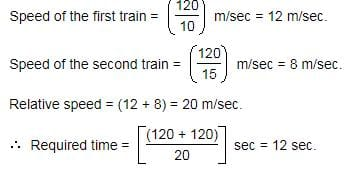Test: Problems On Trains- 1 - Question 6

A train is traveling at 48 kmph . It crosses another train having half of its length , traveling in opposite direction at 42 kmph, in 12 seconds. It also passes a railway platform in 45 seconds. What is the length of the platform?

Detailed Solution for Test: Problems On Trains- 1 - Question 6

► Let the length of the train traveling at 48 kmph be 2x meters.

► And length of the platform is y meters.

► Relative speed of train = (48+42) kmph

= (90*5/18) = 25 m/sec;

And 48 kmph = 48*5/18 = 40/3 m/sec.

► According to the question,

(2x +x)/25 = 12;

Or, 3x = 12*25 = 300;

Or, x = 300/3 = 100m

► Then, length of the train = 2x = 100*2 = 200m.

200+y/(40/3) = 45;

600+3y = 40*45;

Or, 3y = 1800-600 = 1200;

Or, y = 1200/3 = 400 m.

► Length of the platform = 400 m.

Test: Problems On Trains- 1 - Question 7

Two trains, each 100 m long are moving in opposite directions. They cross each other in 8 seconds. If one is moving twice as fast the other, the speed of the faster train is

Detailed Solution for Test: Problems On Trains- 1 - Question 7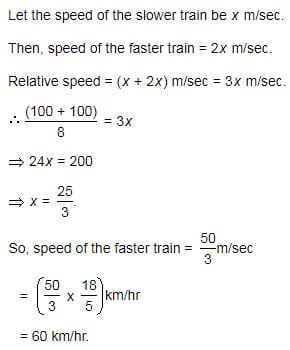Test: Problems On Trains- 1 - Question 8

A 270 metres long train running at the speed of 120 kmph crosses another train running in opposite direction at the speed of 80 kmph in 9 seconds. What is the length of the other train?

Detailed Solution for Test: Problems On Trains- 1 - Question 8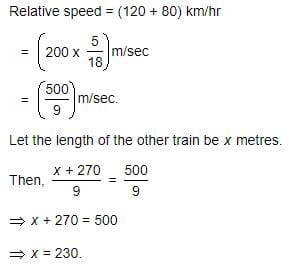Test: Problems On Trains- 1 - Question 9

A train passes a platform in 36 seconds. The same train passes a man standing on the platform in 20 seconds. If the speed of the train is 54 km/hr, The length of the platform is:

Detailed Solution for Test: Problems On Trains- 1 - Question 9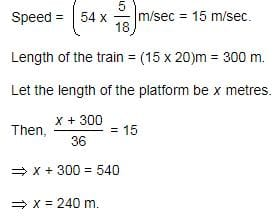Test: Problems On Trains- 1 - Question 10

A train having a length of 1/4 mile , is traveling at a speed of 75 mph. It enters a tunnel 3 ½ miles long. How long does it take the train to pass through the tunnel from the moment the front enters to the moment the rear emerges?

Detailed Solution for Test: Problems On Trains- 1 - Question 10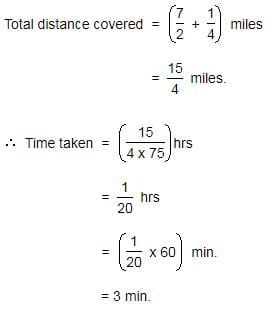## Quantitative Aptitude (Quant)

163 videos|152 docs|131 tests
 Use Code STAYHOME200 and get INR 200 additional OFF Use Coupon Code
Information about Test: Problems On Trains- 1 Page
In this test you can find the Exam questions for Test: Problems On Trains- 1 solved & explained in the simplest way possible. Besides giving Questions and answers for Test: Problems On Trains- 1, EduRev gives you an ample number of Online tests for practice

## Quantitative Aptitude (Quant)

163 videos|152 docs|131 tests

### How to Prepare for CAT

Read our guide to prepare for CAT which is created by Toppers & the best Teachers